# Problem 1. The figure below shows the vectors u, v, and w, along with the images...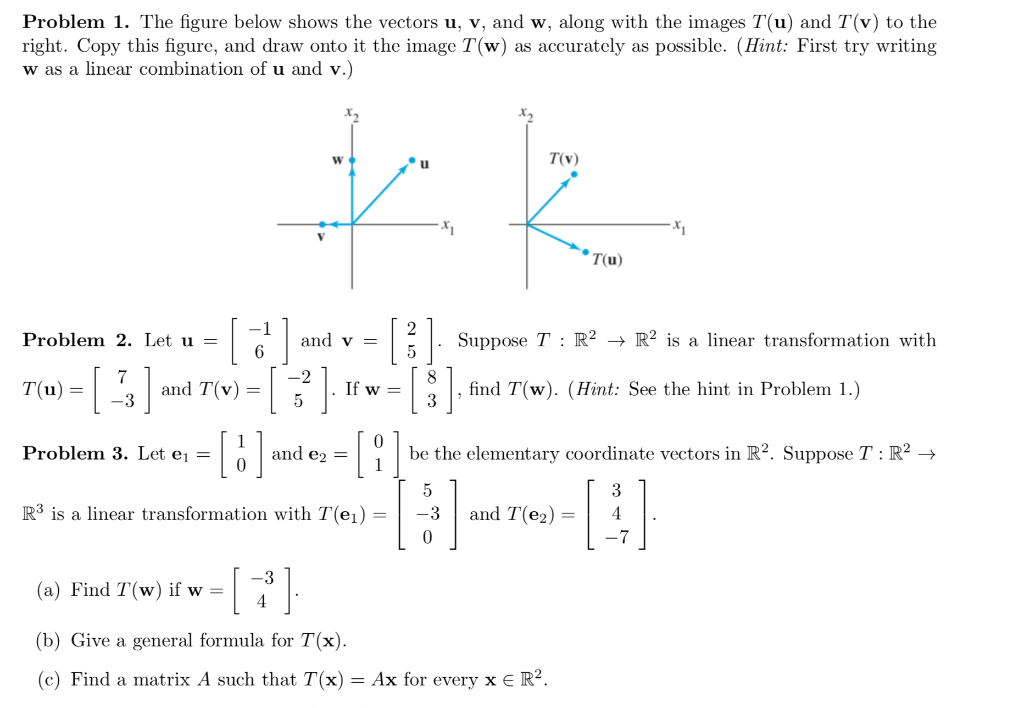Problem 1. The figure below shows the vectors u, v, and w, along with the images T(u) and T(v) to the right. Copy this figure, and draw onto it the image T(w) as accurately as possible. (Hint: First try writing w as a linear combination of u and v.) TV (u) Problem 2. Let u = | and v Suppose T : R2 + R2 is a linear transformation with 6 1 3) Tu = T(u) = -3 and T(v) = an find T(w). (Hint: See the hint in Problem 1.) 1 -3 Problem 3. Let ej = be the elementary coordinate vectors in R2. Suppose T:R2 → ī 5 [ 3 R3 is a linear transformation with T(ei) = | -3 and T(e2) = | 4 L0 -7 - 3 (a) Find T(w) if w = (b) Give a general formula for T(x). (c) Find a matrix A such that T(x) = Ax for every x € R2.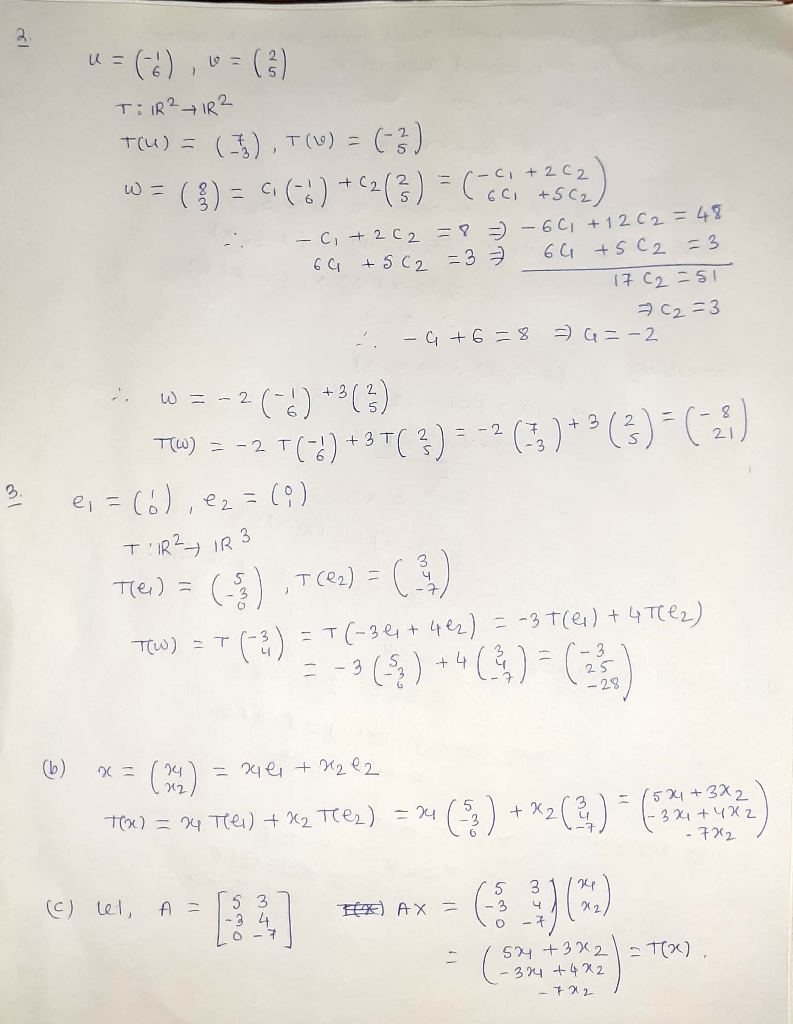##### Add Answer of: Problem 1. The figure below shows the vectors u, v, and w, along with the images...
Similar Homework Help Questions
• ### Let u and v be the vectors shown in the figure to the right, and suppose...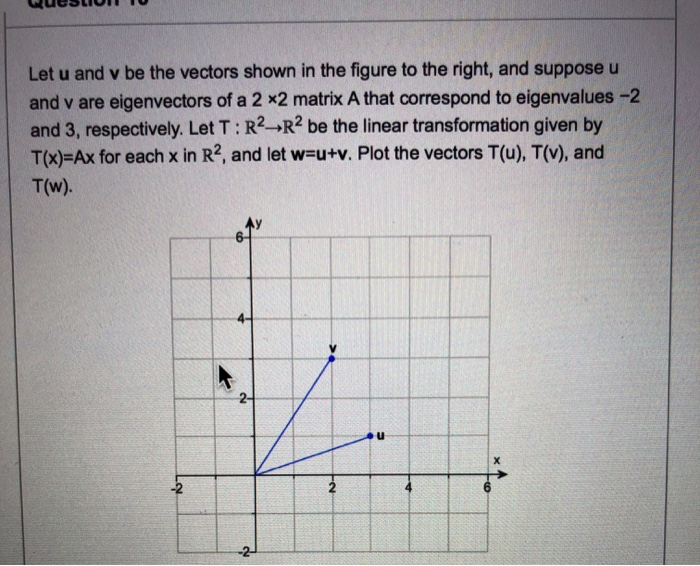Let u and v be the vectors shown in the figure to the right, and suppose u and v are eigenvectors of a 2 x2 matrix A that correspond to eigenvalues -2 and 3, respectively. Let T: R2 R2 be the linear transformation given by T(x)-Ax for each x in R2, and let w-u+v. Plot the vectors T(u), T(v), and T(w). 2- u -2 2 4 -2 10- T(v) T(w -10 10 T(u) -10- Ay 10- T(v) T(w) T(u) 10...

• ### Suppose T: ℝ3→ℝ2 is a linear transformation. Let U and V be the vectors given below, and suppose that T(U) and T(V) are...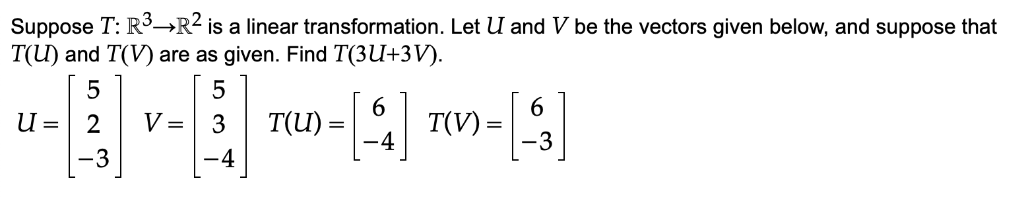Suppose T: ℝ3→ℝ2 is a linear transformation. Let U and V be the vectors given below, and suppose that T(U) and T(V) are as given. Find T(3U+3V). Suppose T: R->R2 is a linear transformation. Let U and V be the vectors given below, and suppose that T(U) and T(V) are as given. Find T(3U+3V). 5 5 6 T(V) 6 =n 2 -3 T(U) V = 3 -4 3 -4 Suppose T: R->R2 is a linear transformation. Let U and V...

• ### Problem 4. Let V be a vector space and let T : V → V and U : V → V be two linear transforinations...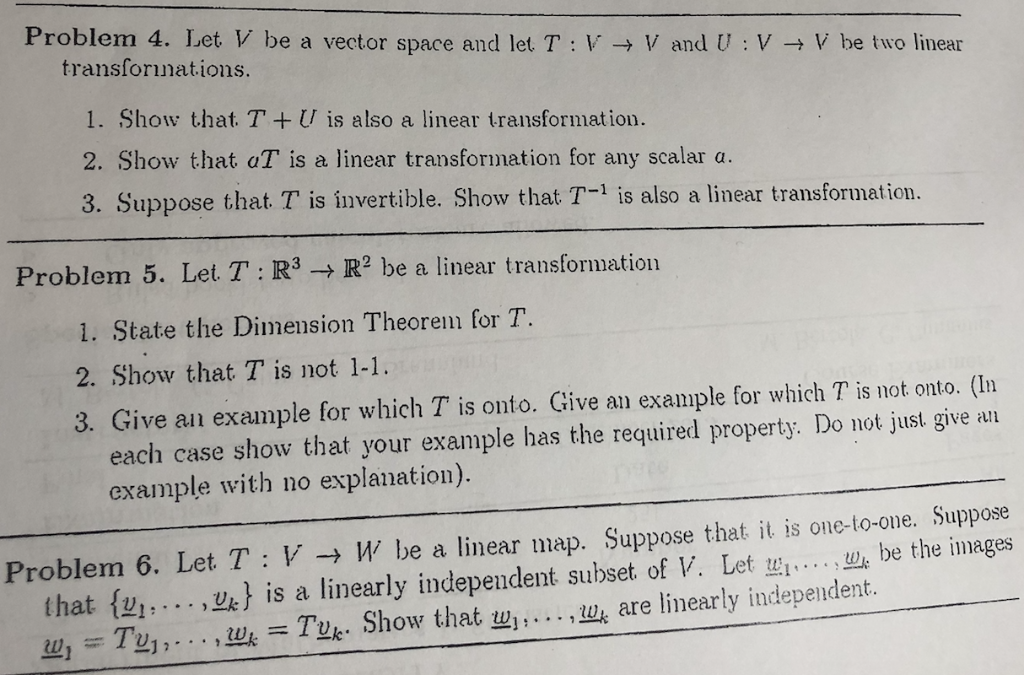I need the answer to problem 6 Clear and step by step please Problem 4. Let V be a vector space and let T : V → V and U : V → V be two linear transforinations 1. Show that. TU is also a linear transformation. 2. Show that aT is a linear transformation for any scalar a. 3. Suppose that T is invertible. Show that T-1 is also a linear transformation. Problem 5. Let T : R3 →...

• ### I need the answer to problem 4 (exercises 1, 2, 3) Clear and step by step...I need the answer to problem 4 (exercises 1, 2, 3) Clear and step by step please Problem 4. Let V be a vector space and let T : V → V and U : V → V be two linear transforinations 1. Show that. TU is also a linear transformation. 2. Show that aT is a linear transformation for any scalar a. 3. Suppose that T is invertible. Show that T-1 is also a linear transformation. Problem 5. Let...

• ### Problem 6 A bilinear pairing on R2 is given on basis vectors by <ei, ei >=...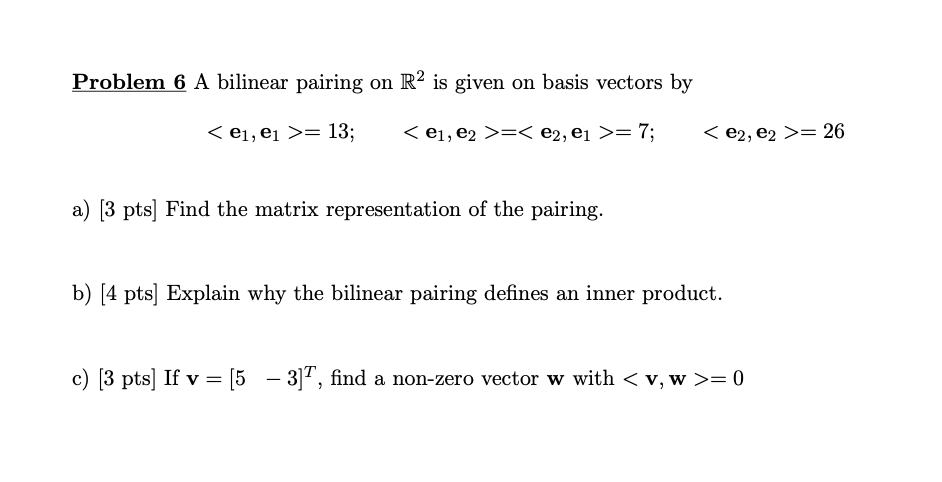Problem 6 A bilinear pairing on R2 is given on basis vectors by <ei, ei >= 13; <ei, e2 >=< e2, ej >= 7; <e2,e2 >= 26 a) [3 pts) Find the matrix representation of the pairing. b) (4 pts) Explain why the bilinear pairing defines an inner product. c) [3 pts) If v = [5 – 3]T, find a non-zero vector w with < v, w >= 0

• ### Vectors : If u and v are vectors such that..

|u|=2 , |v| = 3 and the angle between u and v is pi/3a)Find the exact value for |u - 2v|b) let u = <4,-8,1> , v = <2,1,-2> and w = <3,-4,12> determine if the vectors u,v and w are coplanar. Justify your answerc) Let u,v and w be vectors such that u . (v x w) = 3 Find(i) u.(w x v) (ii) (u x w).v (iii) v.(w x w)

• ### Thank you Find u. (w). This quantity is called the triple scalar product of u, v,...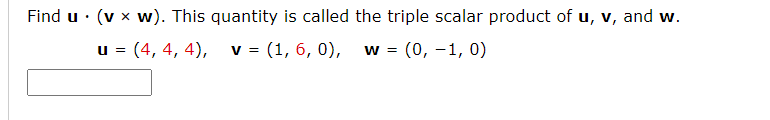Thank you Find u. (w). This quantity is called the triple scalar product of u, v, and w. u = (4, 4, 4), v = (1, 6, 0), (0, -1,0) W = Let T: R3 R3 be a linear transformation such that T(1, 1, 1) = (4,0, -1), T(0, -1, 2) = (-5,2, -1), and T(1, 0, 1) = (1, 1, 0). Find the indicated image. T(2, -1, 1) T(2, -1, 1) = Let T be a linear transformation from...

• ### Let V be an inner product space and u, w be fixed vectors in V . Show that T v = <v, u>w defines a linear operator...

Let V be an inner product space and u, w be fixed vectors in V . Show that T v = <v, u>w defines a linear operator in V . Show that T has an adjoint, and describe T ∗ explicitly

• ### Please answer me fully with the details. Thanks! Let V and W be vector spaces, let B = (j,...,Tn) be a basis of V, and...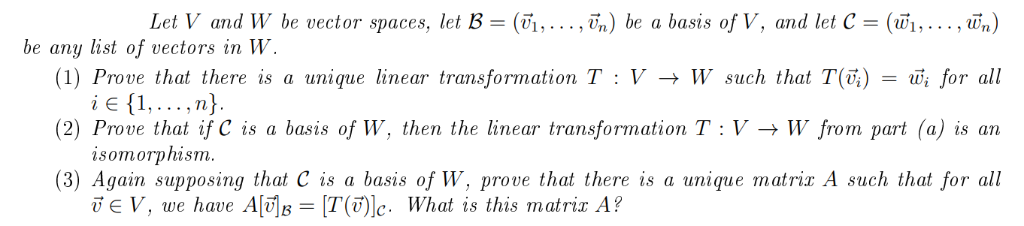Please answer me fully with the details. Thanks! Let V and W be vector spaces, let B = (j,...,Tn) be a basis of V, and let C = (Wj,..., Wn) be any list of vectors in W. (1) Prove that there is a unique linear transformation T : V -> W such that T(V;) i E 1,... ,n} (2) Prove that if C is a basis of W, then the linear transformation T : V -> W from part (a)...

• ### (6 marks) Suppose that u, v and w are vectors in R3, and that u. (v...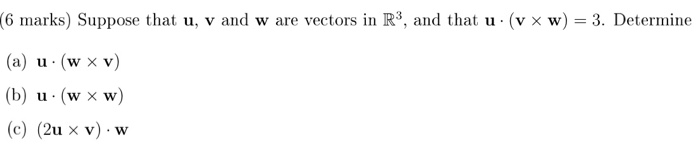(6 marks) Suppose that u, v and w are vectors in R3, and that u. (v x w) = 3. Determine (a) u (w xv) (b) u. (w xw) (c) (2u x v). w

Free Homework App